## LetsPlayMaths.Com

WELCOME TO THE WORLD OF MATHEMATICS

# Class 3 Fraction

Introduction to Fractions

Numerator and Denominator

Equivalent Fractions

Properties of Equivalent Fractions

Comparison of Fractions

Addition of Fractions

Subtraction of Fractions

Fraction Test

Fraction Worksheet

Answer Sheet

## Introduction to Fractions

Let’s consider a circle as shown in the figure below.It is divided into two equal parts where one part is shaded with black. The shaded part is one half of the whole circle. We express one half by the symbol 1/2. We read it as one by two. The unshaded part is also 1/2 of the circle.

Here the circle is divided into 4 equal parts as shown in the figure below.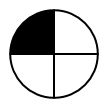One part of the circle is shaded with black. The shaded portion is 1/4 of the whole circle. We read it as one by four.

Here the circle is divided into 8 equal parts as shown in the figure below.One part of the circle is shaded with black. The shaded portion is 1/8 of the whole circle. We read it as one by eight.

Here the circle is divided into 8 equal parts as shown in the figure below.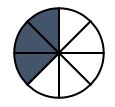Three parts of it are shaded. The shaded portion is 3/8 of the whole circle. We read it as three by eight.

So, 1/2, 1/4, 1/8 and 3/8 are known as fractions.

## Numerator and Denominator

We have learnt that a fraction is written with two numerals arranged one over the other and are separated by a line “/”. The number which is present at the right side of the line shows in how many equal parts the whole has been divided into. It is called Denominator of the fraction. The number which is present at the left side of the line shows how many parts of the whole have been taken. It is called Numerator of the fraction.

So, in 2/3, 2 is the numerator and 3 is the denominator.

## Equivalent Fractions

Let us look at the following circles of the same size.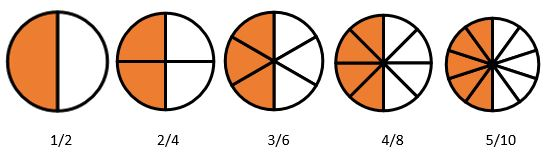The shaded parts in each figure are same.

1/2, 2/4, 3/6, 4/8 and 5/10 represent equal shaded parts.

Hence, we can say that 1/2 = 2/4 = 3/6 = 4/8 = 5/10

We say 1/2, 2/4, 3/6, 4/8 and 5/10 are equivalent fractions.

## Properties of Equivalent Fractions

1. If we multiply the numerator and denominator of a fraction by the same number (Except zero) the value of the fraction does not change.

Example.

1/2 X 1/1 = 1/2   1/2 X 2/2 = 1/2
1/2 X 3/3 = 3/6   1/2 X 4/4 = 4/8

2. If we divide the numerator and denominator of a fraction by the same number (Except zero) the value of the fraction dies not change.

Example.

1/2 ÷ 1/1 = 1/2   1/2 ÷ 2/2 = 1/2
1/2 ÷ 3/3 = 1/2   1/2 ÷ 4/4 = 1/2

## Comparison of FractionsThere are 5 similar strips. The shaded portion of the first strip is more than the shaded portion of the second strip. Therefore, 1 > 1/2.

The shaded portion of the second ship is more than the shaded portion of the third strip. Therefore, 1/2 > 1/3

Similarly, 1/3 > 1/4, 1/4 > 1/5, 1/5 > 1/6, 1/6 > 1/7, 1/7 > 1/8, 1/8 > 1/9, 1/9 > 1/10

Let us consider some other example.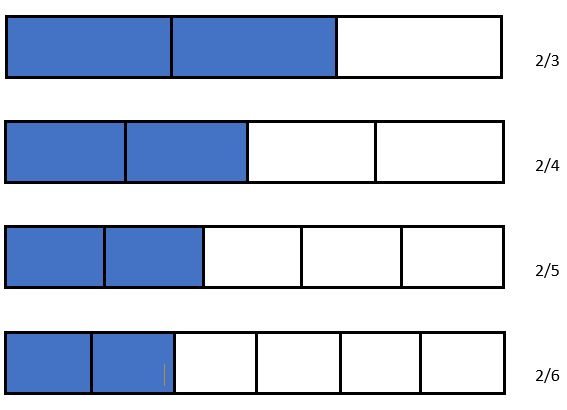Similarly, from the above example we can say, 2/3 > 2/4, 2/4 > 2/5, 2/5 > 2/6

From the above two examples we conclude that, if two fractions have same numerator the fraction having smaller denominator is greater than the other.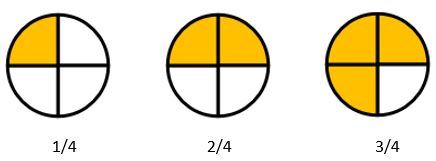From the shaded portion in the circles above, it is clear that 2/4 > 1/4 and 3/4 > 2/4.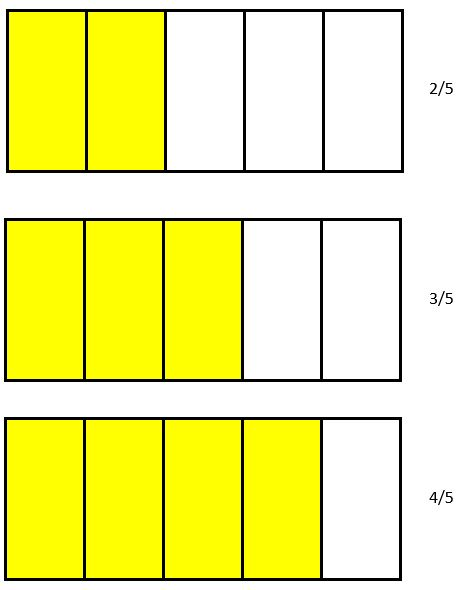From the shaded portions in the above figures, we can say that
2/5 < 3/5 and 3/5 < 4/5
So, from the above two examples we can conclude that if two fractions have the same denominator, the one with the greater numerator is greater than the other.

Example 1. Which is greater, 3/5 or 7/5?

Solution. Both the fractions have the same denominator but (numerator) 7 > (numerator) 3
So, 7/5 > 3/5

Example 2. Which is smaller, 4/11 or 4/13?

Solution. Both the fractions have same numerator but (denominator) 11 < (denominator) 13
So, 4/13 < 4/11

## Addition of Fractions

Let’s consider a rectangle and divide it into 8 equal parts. Three parts on the left and one part on the right of the rectangle are shaded.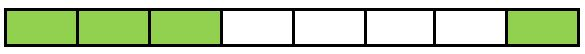Three shaded parts represents 3/8 of the whole and 1 shaded part represent 1/8 of the whole.

When both the shaded parts taken together, it represents 4/8.

So, 3/8 + 1/8 = (3+1)/8 = 4/8

Similarly, let’s take another example of a circle.Left shaded part of the circle represents 1/6 of the whole.

Right shaded part of the circle represents 3/6.

When both the shaded parts taken together, it represents 4/6

1/6 + 3/6 = (1+3)/6 = 4/6

From the above two examples, we can derive that the sum of two or more fractions having the same denominator is Sum of Numerators / Denominator.

## Subtraction of Fractions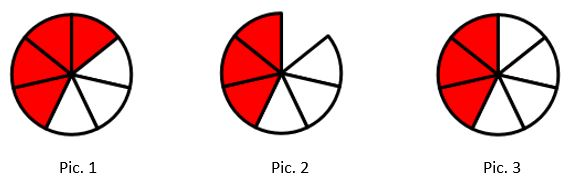In Pic. 1 circle is divided into 7 equal parts, out of which 4 parts are shaded. The shaded portions represent as 4/7.

In Pic. 2 one shaded part is removed, it is represented as 1/7.

In Pic. 3 remaining shaded part is shown, and it represent as 3/7.

4/7 – 1/7 = (4 - 1) / 7 = 3/7

## Fraction Test

Fraction Test - 1

Fraction Test - 2

Fraction Test - 3

## Fraction Worksheet

Fraction Worksheet - 1

Fraction Worksheet - 2

Fraction Worksheet - 3

Fraction Worksheet - 4

## Answer Sheet

Fraction-AnswerDownload the pdf

Copyright © 2021 LetsPlayMaths.com. All Rights Reserved.